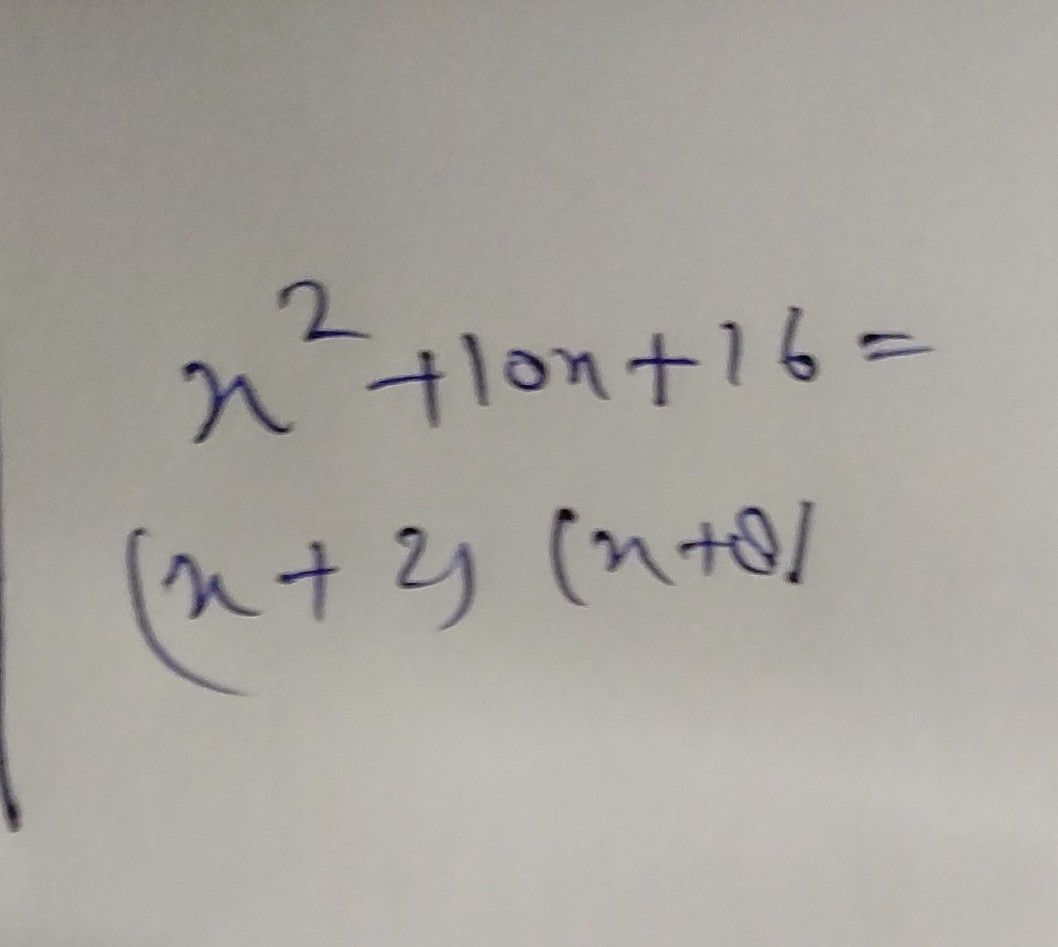Symbol
Problem$x^{2}+10x+15=|$ $\left($ $x-2$ $x-1$ $x^{-+}$ $x+1\square$ $x+5$